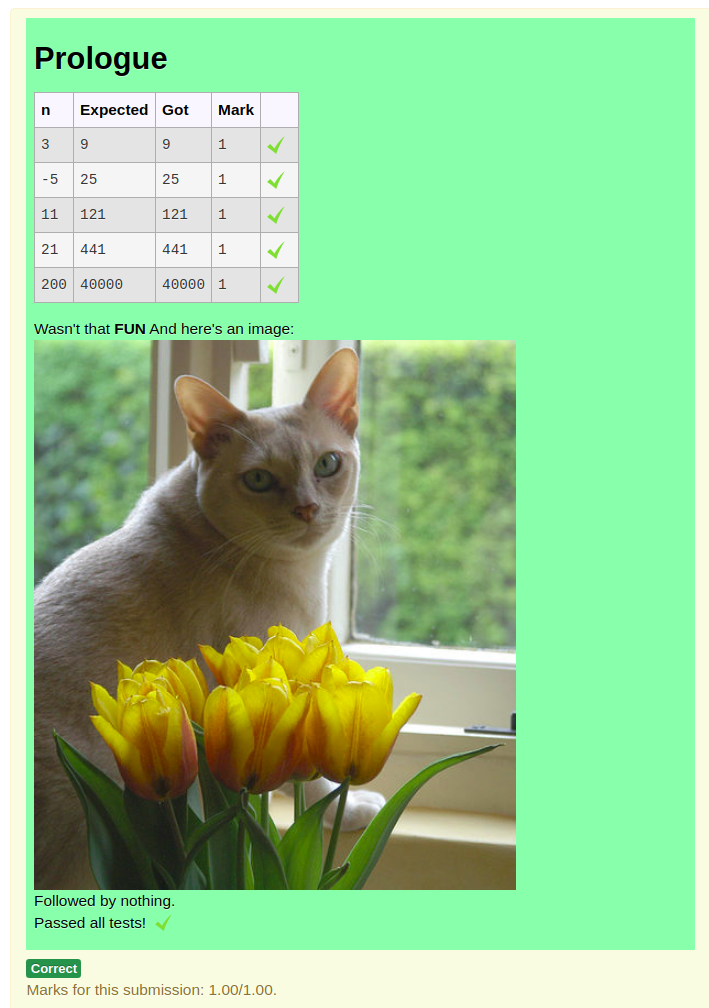Developers' Forum

Octave graphicsRe: Octave graphics

de Richard Lobb -
Número de respuestas: 0

It's possible to return and display images, but it's not easy. You need to use a combinator template grader in which either the prologue or epilogue HTML is a data-url containing the image to be displayed.

I attach a combinator-template grader example that displays an image. Here's its template:

import json
import base64

def get_jpeg_b64(filename):
"""Return the contents of the given file (assumed to be jpeg) as a base64
encoded string in utf-8.
"""
with open(filename, 'br') as fin:

return base64.b64encode(contents).decode('utf8')

# First, build a standard result table from testing the student sqr code.
# To be safe this should be done in a subprocess that catches any errors
# but this is just a simple demo. So we assume no errors.
n_vals = [3, -5, 11, 21, 200];
results = [['n', 'Expected', 'Got', 'Mark', 'IsCorrect']]
total = 0
for n in n_vals:
got = sqr(n)
mark = 1 if got == n * n else 0
total += mark
results.append([n, n * n, got, mark, got == n * n])

# Now get a data-url containing the contents of an image, which in this case
# was included in the uploaded support files, again just as a demo.
filename = 'catimage.jpeg'
image_data = get_jpeg_b64(filename)
html = """Wasn't that <b>FUN</b>
And here's an image:<br>
<img class="data-uri-example" title="Jinny by the Tulips" src="data:image/jpeg;base64,{}" alt="{}">
<br>Followed by nothing.""".format(image_data, filename)

# Lastly return the JSON-encoded result required by a combinator grader
print(json.dumps({'prologuehtml': "<h2>Prologue</h2>",
'testresults': results,
'epiloguehtml': html,
'fraction': total / len(n_vals)
}))

And here's the output:I haven't myself used images in production questions, although a graphics lecturer at the University of Auckland, Dr Burkhard Wuensche, has made extensive use of this technique. He runs the student code (which uses OpenGL) in a C++ subprocess. You would need to run the Octave code in a subprocess, too. When using combinator template graders it is essential to run student code in a subprocess because the grader is required to always return valid JSON. If you don't use a subprocess (with time limit control) any errors in the student code will result in an invalid return value from the grader.

But ... the real problem with images is figuring out how to grade them.

Richard